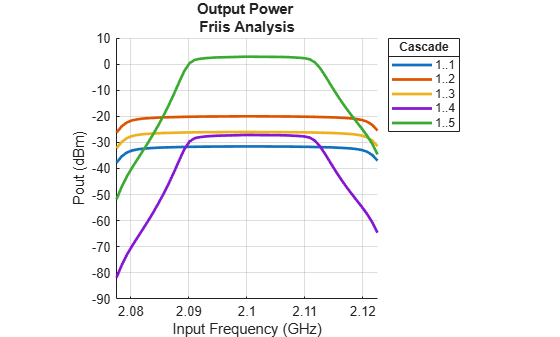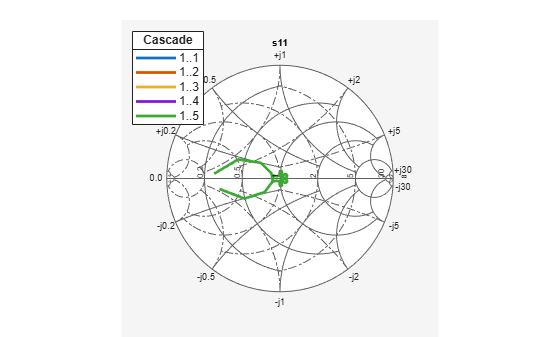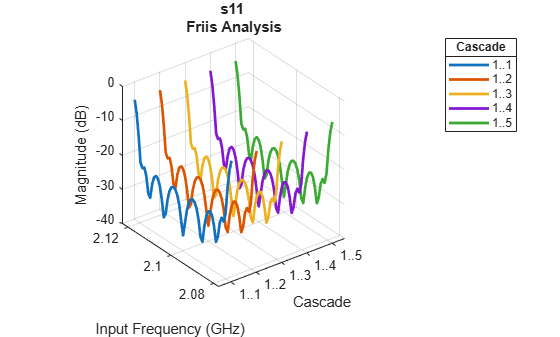# rfbudget

Create RF budget object and compute RF budget results for chain of 2-port elements

## Description

Use the `rfbudget` object to create an RF budget object and compute the RF budget results for a chain of 2-port elements. In this RF chain, you can use a 2-port element such as `amplifier`, `nport`, or `modulator`. You can also open the `rfbudget` object in an RF Budget Analyzer app and then export the completed circuit to RF Blockset™ for circuit envelope analysis.

## Creation

### Syntax

``rfobj = rfbudget``
``rfobj = rfbudget(elements,inputfreq,inputpwr,bandwidth)``
``rfobj = rfbudget(___,autoupdate)``
``rfobj = rfbudget(Name=value)``

### Description

example

````rfobj = rfbudget` creates an `rfbudget` object, `rfobj`, with default empty property values.```

example

````rfobj = rfbudget(elements,inputfreq,inputpwr,bandwidth)` sets Elements, InputFrequency, AvailableInputPower, and SignalBandwidth properties and computes the RF budget analysis. By default, if any of the input properties are changed, the object recomputes results.```
````rfobj = rfbudget(___,autoupdate)` sets the AutoUpdate property. You can use this syntax with any of the previous syntaxes.```

example

````rfobj = rfbudget(Name=value)` sets Properties using one or more name-value arguments. You can specify multiple name-value arguments.```

## Properties

expand all

RF budget elements, specified as an RF budget object or an array of RF budget objects. Use an array of RF budget objects when you perform RF budget analysis on an RF chain.

This table lists supported RF budget elements you can use to design an RF chain.

Example: ```a = amplifier; m = modulator; rfbudget(Elements=[a m])``` calculates the RF budget analysis of the amplifier and modulator circuit.

Input frequency of the signal, specified as a scalar or column vector of size M-by-1 in Hz. M represents number of frequencies. If the input frequency is a vector, then the RF budget object analyzes each input frequency separately.

Example: `InputFrequency=2e9`

Data Types: `double`

Power applied at the input of the cascade, specified as a scalar in dBm.

Example: `AvailableInputPower=-30`

Data Types: `double`

Signal bandwidth at the input of the cascade, specified as a scalar in Hz.

Example: `SignalBandwidth=10`

Data Types: `double`

Automatically recompute the RF budget analysis by incorporating changes made to the existing circuit, specified as `true` or `false`.

Setting `AutoUpdate` to `false` turns off automatic budget recomputation as parameters change. To compute the budget result of an `rfbudget` object when you set the `AutoUpdate` property to `false`, use the `computeBudget` function.

Example: `AutoUpdate=false`

Data Types: `logical`

Computation method, specified as `Friis` or `HarmonicBalance`. The `Friis` solver is faster and the `HarmonicBalance` solver supports computation of second-order nonlinearities such as OIP2.

When you set the `Solver` type to `HarmonicBalance`, the tone and harmonic-dependent properties are displayed.

Note

The `HarmonicBalance` solver does not support architectures where the input or output frequencies at any stage in the cascade are nonzero and less than SignalBandwidth.

Example: `Solver='Friis'`

Data Types: `string`

Number of harmonics to use for one-tone harmonic balance (HB) analysis, specified as a positive integer. For each two-tone analysis, `max(3,HarmonicOrder)` harmonics is used. Use the default value for automatic determination of harmonics.

Use this property to

• Accelerate the HB analysis by reducing the number of harmonics needed for a mildly nonlinear system.

• Ensure harmonic balance accuracy by increasing the number of harmonics used in a highly nonlinear system.

#### Dependencies

To enable this property, set Solver to `HarmonicBalance`.

Data Types: `double`

Output frequencies in Hz, returned as one of the following:

• Scalar when M and N = `1`

• Vector when M or N = `1`

• Matrix when M and N > `1`

where M represents the number of frequencies in the input and N represents the number of stages in the cascade.

Data Types: `double`

Output power in dBm, returned as one of the following:

• Scalar when M and N = `1`

• Vector when M or N = `1`

• Matrix when M and N > `1`

where M represents the number of frequencies in the input and N represents the number of stages in the cascade.

Data Types: `double`

Transducer power gains in dB, returned as one of the following:

• Scalar when M and N = `1`

• Vector when M or N = `1`

• Matrix when M and N > `1`

where M represents the number of frequencies in the input and N represents the number of stages in the cascade.

Data Types: `double`

Noise figures in dB, returned as one of the following:

• Scalar when M and N = `1`

• Vector when M or N = `1`

• Matrix when M and N > `1`

where M represents the number of frequencies in the input and N represents the number of stages in the cascade.

Note

If AvailableInputPower is very large, it can result in negative `NF` values during harmonic balance analysis .

Data Types: `double`

Input-referred second-order intercept (IIP2) in dBm, returned as one of the following:

• Scalar when M and N = `1`

• Vector when M or N = `1`

• Matrix when M and N > `1`

where M represents the number of frequencies in the input and N represents the number of stages in the cascade.

#### Dependencies

To compute IIP2 values, set Solver to `HarmonicBalance`.

Data Types: `double`

Output-referred second-order intercept (OIP2) in dBm, returned as one of the following:

• Scalar when M and N = `1`

• Vector when M or N = `1`

• Matrix when M and N > `1`

where M represents the number of frequencies in the input and N represents the number of stages in the cascade.

#### Dependencies

To compute OIP2 values, set Solver to `HarmonicBalance`.

Data Types: `double`

The Input-referred third-order intercept (IIP3) in dBm, returned as one of the following:

• Scalar when M and N = `1`

• Vector when M or N = `1`

• Matrix when M and N > `1`

where M represents the number of frequencies in the input and N represents the number of stages in the cascade.

Data Types: `double`

The Output-referred third-order intercept (OIP3) in dBm, returned as one of the following:

• Scalar when M and N = `1`

• Vector when M or N = `1`

• Matrix when M and N > `1`

where M represents the number of frequencies in the input and N represents the number of stages in the cascade.

Data Types: `double`

Signal-to-noise ratio (SNR) in dB, returned as one of the following:

• Scalar when M and N = `1`

• Vector when M or N = `1`

• Matrix when M and N > `1`

where M represents the number of frequencies in the input and N represents the number of stages in the cascade.

Data Types: `double`

Display a progress bar with a cancel button during harmonic balance analysis, specified as `true` or `false`.

Data Types: `logical`

## Object Functions

 `show` Display RF budget object in RF Budget Analyzer app `computeBudget` Compute results of RF budget object `exportScript` Export MATLAB code that generates RF budget object `exportRFBlockset` Create RF Blockset model from RF budget object `exportTestbench` Create measurement testbench from RF budget object `rfplot` Plot cumulative RF budget result versus cascade input frequency `smithplot` Plot measurement data on Smith chart `polar` Plot specified object parameters on polar coordinates

## Examples

collapse all

Open a default RF budget object.

`obj = rfbudget`
```obj = rfbudget with properties: Elements: [] InputFrequency: [] Hz AvailableInputPower: [] dBm SignalBandwidth: [] Hz Solver: Friis AutoUpdate: true ```

Create an amplifier with a gain of 4 dB.

`a = amplifier(Gain=4);`

Create a modulator with an OIP3 of 13 dBm.

`m = modulator(OIP3=13);`

Create an N-port element using `passive.s2p`.

`n = nport('passive.s2p');`

Create an RF element with a gain of 10 dB.

`r = rfelement(Gain=10);`

Calculate the RF budget of a series of RF elements at an input frequency of 2.1 GHz, an available input power of –30 dBm, and a bandwidth of 10 MHz.

`b = rfbudget([a m r n],2.1e9,-30,10e6)`
```b = rfbudget with properties: Elements: [1x4 rf.internal.rfbudget.Element] InputFrequency: 2.1 GHz AvailableInputPower: -30 dBm SignalBandwidth: 10 MHz Solver: Friis AutoUpdate: true Analysis Results OutputFrequency: (GHz) [ 2.1 3.1 3.1 3.1] OutputPower: (dBm) [ -26 -26 -16 -20.6] TransducerGain: (dB) [ 4 4 14 9.4] NF: (dB) [ 0 0 0 0.1392] IIP2: (dBm) [] OIP2: (dBm) [] IIP3: (dBm) [ Inf 9 9 9] OIP3: (dBm) [ Inf 13 23 18.4] SNR: (dB) [73.98 73.98 73.98 73.84] ```

Type the `show` command at the command window to display the analysis in the RF Budget Analyzer app.

```show(b) ```Create an RF system.

Create an RF bandpass filter using the Touchstone® file `RFBudget_RF`.

`f1 = nport('RFBudget_RF.s2p','RFBandpassFilter');`

Create an amplifier with a gain of 11.53 dB, a noise figure (NF) of 1.53 dB, and an output third-order intercept (OIP3) of 35 dBm.

`a1 = amplifier(Name='RFAmplifier',Gain=11.53,NF=1.53,OIP3=35);`

Create a demodulator with a gain of –6 dB, a NF of 4 dB, and an OIP3 of 50 dBm.

```d = modulator(Name='Demodulator',Gain=-6,NF=4,OIP3=50, ... LO=2.03e9,ConverterType='Down');```

Create an IF bandpass filter using the Touchstone file `RFBudget_IF`.

`f2 = nport('RFBudget_IF.s2p','IFBandpassFilter');`

Create an amplifier with a gain of 30 dB, a NF of 8 dB, and an OIP3 of 37 dBm.

`a2 = amplifier(Name='IFAmplifier',Gain=30,NF=8,OIP3=37);`

Calculate the RF budget of the system using an input frequency of 2.1 GHz, an input power of –30 dBm, and a bandwidth of 45 MHz.

`b = rfbudget([f1 a1 d f2 a2],2.1e9,-30,45e6)`
```b = rfbudget with properties: Elements: [1x5 rf.internal.rfbudget.Element] InputFrequency: 2.1 GHz AvailableInputPower: -30 dBm SignalBandwidth: 45 MHz Solver: Friis AutoUpdate: true Analysis Results OutputFrequency: (GHz) [ 2.1 2.1 0.07 0.07 0.07] OutputPower: (dBm) [-31.53 -20 -26 -27.15 2.847] TransducerGain: (dB) [-1.534 9.996 3.996 2.847 32.85] NF: (dB) [ 1.533 3.064 3.377 3.611 7.036] IIP2: (dBm) [] OIP2: (dBm) [] IIP3: (dBm) [ Inf 25 24.97 24.97 4.116] OIP3: (dBm) [ Inf 35 28.97 27.82 36.96] SNR: (dB) [ 65.91 64.38 64.07 63.83 60.41] ```

Plot the available output power.

```rfplot(b,'Pout') view(90,0)```Plot the transducer gain.

```rfplot(b,'GainT') view(90,0)```Plot S-parameters of an RF system on a Smith Chart and a Polar plot.

`s = smithplot(b,1,1,'GridType','ZY'); ``p = polar(b,2,1); `Create two modulators with output-referred second-order intercept set to 20 and available power gain set to 3.

```m = modulator(Gain=3,OIP2=20,ImageReject=false,ChannelSelect=false); m2 = modulator(Gain=3,OIP2=20,ImageReject=false,ChannelSelect=false);```

Create an RF budget object specifying the input frequency of the signal, power applied at cascade, and signal bandwidth. Select `HarmonicBalance` as solver method to compute nonlinear effects such as IIP2 and OIP2.

`b = rfbudget([m m2],2.1e9,-30,100e6,Solver='HarmonicBalance')`
```b = rfbudget with properties: Elements: [1x2 modulator] InputFrequency: 2.1 GHz AvailableInputPower: -30 dBm SignalBandwidth: 100 MHz Solver: HarmonicBalance WaitBar: true AutoUpdate: true Analysis Results OutputFrequency: (GHz) [ 3.1 4.1] OutputPower: (dBm) [ -27 -24] TransducerGain: (dB) [ 3 6] NF: (dB) [ 3.01 7.783] IIP2: (dBm) [ 17 4.457] OIP2: (dBm) [ 20 10.46] IIP3: (dBm) [ Inf Inf] OIP3: (dBm) [ Inf Inf] SNR: (dB) [60.96 56.19] ```

Create an amplifier with a gain of 10 dB.

`a = amplifier(Gain=10);`

Create a modulator with an OIP3 of 13 dBm.

`m = modulator(OIP3=13);`

Create an N-port circuit element using `passive.s2p`.

`n = nport('passive.s2p');`

Calculate the RF budget of a series of RF elements at an input frequency of 2.1 GHz, an available input power of –30 dBm, and a bandwidth of 10 MHz using HB analysis. Set the number of harmonics that the `rfbudget` object should use for all the tones in HB analyses.

```b = rfbudget([a m n],2.1e9,-30,10e6,... Solver="HarmonicBalance",HarmonicOrder=3)```
```b = rfbudget with properties: Elements: [1x3 rf.internal.rfbudget.Element] InputFrequency: 2.1 GHz AvailableInputPower: -30 dBm SignalBandwidth: 10 MHz Solver: HarmonicBalance HarmonicOrder: 3 WaitBar: true AutoUpdate: true Analysis Results OutputFrequency: (GHz) [ 2.1 3.1 3.1] OutputPower: (dBm) [ -20 -20 -24.6] TransducerGain: (dB) [ 10 9.996 5.396] NF: (dB) [-2.842e-14 -0.004353 0.3376] IIP2: (dBm) [ Inf Inf Inf] OIP2: (dBm) [ Inf Inf Inf] IIP3: (dBm) [ Inf 2.993 2.995] OIP3: (dBm) [ Inf 12.98 8.382] SNR: (dB) [ 73.98 73.98 73.64] ```

Create an amplifier with a gain of 4 dB.

`a = amplifier(Gain=4);`

Create a modulator with an OIP3 of 13 dBm.

`m = modulator(OIP3=13);`

Create an N-port element using `passive.s2p`.

`n = nport('passive.s2p');`

Create an RF element with a gain of 10 dB.

`r = rfelement(Gain=10);`

Calculate the RF budget of a series of RF elements at an input frequency of 2.1 GHz, an available input power of –30 dB, and a bandwidth of 10 MHz.

`b = rfbudget([a m r n],2.1e9,-30,10e6);`

Show the analysis in the RF plot.

`rfplot(b)`Group Delay

To plot the group delay, first plot the S11 data for the RF System.

`rfplot(b,1,1)`Use the `Group Delay `option on the plot graph to plot the group delay of the RF system.Phase Delay

Use the `Phase Delay` option on the plot graph to plot the phase delay of the RF System.## Algorithms

expand all

ABCD parameters are used in the computation of S-parameters of the cascade for Friis Solver. When S21 = 0, conversion to ABCD results in NaNs. For such cases, modifications to the S-parameters are made as follows:

 Roychowdhury, J., D. Long, and P. Feldmann. “Cyclostationary Noise Analysis of Large RF Circuits with Multitone Excitations.” IEEE Journal of Solid-State Circuits 33, no. 3 (March 1998): 324–36. https://doi.org/10.1109/4.661198.